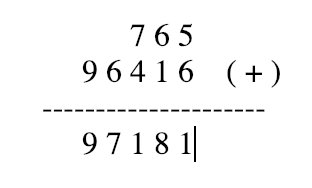Showing posts with label Addition of 2 Linked List. Show all posts
Showing posts with label Addition of 2 Linked List. Show all posts## Add two numbers represented by linked lists using recursive

Add two numbers represented by linked lists using recursive method and generate the 3td linked with addition of 1st and 2nd linked list.

For example:

val_1[] = {       7, 6, 5 }
val_2[] = { 9, 6, 4, 1, 6 }

then the output should be {9, 7, 1, 8, 1} basically its addition of 1st and 2nd linked lists as same normal 2 numbers addition.

Let's see simple java code to create linked lists using input array and to get the addition of 2 linked list in 3rd linked list.

```public class SumOfTwoLL {

class NODE {

int data;
NODE next;

public NODE(int data) {
this.data = data;
this.next = null;
}
}

public static void main(String[] args) {

SumOfTwoLL obj = new SumOfTwoLL();

int val_1[] = new int[] {       7, 6, 5 };
int val_2[] = new int[] { 9, 6, 4, 1, 6 };

// Create linked list out of arrays
NODE first = obj.createLinkedList(val_1);
NODE second = obj.createLinkedList(val_2);

// Check no. of nodes in both Linked list
int t1 = obj.noOfNodes(first);
int t2 = obj.noOfNodes(second);

// Adjusting Linked List with leading zero node's to make it equal size
if(t1 < t2) first = obj.adjustLinkedList(first, (t2-t1));
else if(t2 < t1) second = obj.adjustLinkedList(second, (t1-t2));

// add method call
NODE third = obj.addTwoLL(first, second, 0);

obj.print(third);

}

// Method to get sum of 2 Linked List
private NODE addTwoLL(NODE first, NODE second, int cnt) {

if(first != null && second != null){

// Recursive call moving to last node
NODE node = addTwoLL(first.next, second.next, cnt+1);

// Calculating sum from last 2 values
int val = (first.data + second.data) +node.data;

int carry = val/10;
int sum = val%10;

// If carry present or not the first node in list then create a node with carry value
if(cnt != 0 || carry > 0) {

NODE carryNode = new NODE(carry);
node.data = sum;
carryNode.next = node;
return carryNode;

}else {
// Assign final sum to the previous cycle carry node
node.data = sum;
return node;
}
}
// Create last carry node with zero
return new NODE(0);
}

NODE tmp = new NODE(0);
tmp.next = ll;
ll = tmp;
}
return ll;
}

private int noOfNodes(NODE ll){
int count = 0;
while(ll !=  null){
count ++;
ll = ll.next;
}
return count;

}

public NODE createLinkedList(int val[]) {
NODE start = null;
for (int i : val) {
NODE tmp = new NODE(i);
if (start == null) {
start = tmp;
} else {
NODE mover = start;
while (mover.next != null) {
mover = mover.next;
}
mover.next = tmp;
}
}
return start;
}

public void print(NODE tmp){
while(tmp != null ){
System.out.print(tmp.data +", ");
tmp = tmp.next;
}
}
}
```

OUTPUT:

```9, 7, 1, 8, 1,
```